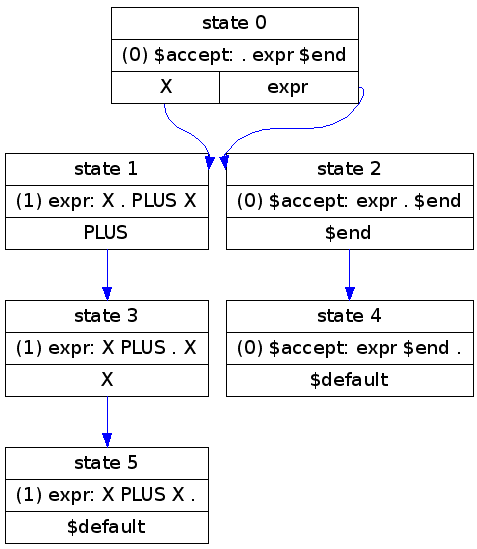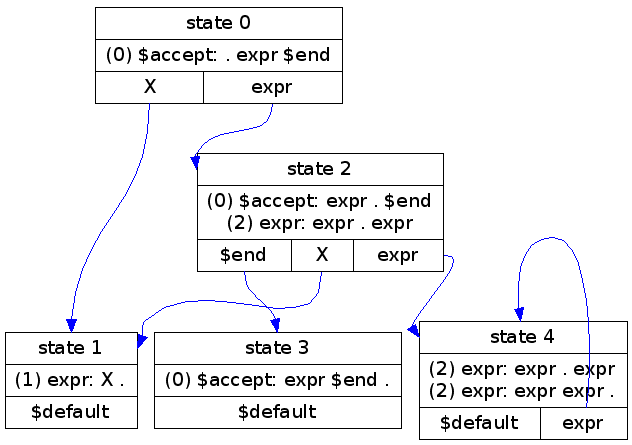# YACC precedence and shift/reduce errors

While I was working on a BibTeX parser, I ran into some trouble with shift/reduce errors in `yacc`. The problem essentially boiled down to: How do you define implicit multiplication in `yacc`?

Keep reading for my intro to `yacc`, or, if you just want the simple solution, skip to the end.

## Related work

After much googling about, I would like to recommend the following sources:

• David Beazley's PLY documentation which has an good introduction to shift/reduce errors with a nice walkthrough shift/reduce example;
• Stephen Johnson's yacc introduction (see Section 4: How the Parser Works), which helped me understand the state-stack concept which hadn't sunk in until then;
• Leon Kaplan's yaccviso documenation, which reminded me that visualizations are good, and inspired my resurrection of the `yacc2dot` tool in Python; and
• Xavier Leroy et al.'s ocamlyacc documentation, where rule-vs-operator precedence finally clicked.

For further details and entertaining browsing, you can take a slow meander through the Bison manual.

## Tools

The following graphics were generated from the `y.output` files output by `yacc` using yacc2dot.py script and Graphviz dot.

## Example 1: Single, explicit addition

As a brief introduction to how parsers work, consider the really simple grammar

which generates the following `yacc` output and `dot` image:Parsing input like `X PLUS X` would look like

StepState stackSymbol stackSymbol queueAction
00\$acceptX PLUS X \$endShift X
101\$accept XPLUS X \$endShift PLUS
2013\$accept X PLUSX \$endShift X
30135\$accept X PLUS X\$endReduce using rule 1, pop back to state 0, and goto state 2
402\$accept expr\$endShift \$end
5024\$accept expr \$endAccept using rule 0

The key step here is 3-to-4. We reduce using Rule 1

``````(1) expr: X PLUS X
``````

Since there are three symbols on the rule's right hand side, we pop three symbols from the stack. The symbols we pop match the right hand side, which is why we can apply the rule. We also pop three states (1, 3, and 5), which returns us to state 0. We look up state 0's action for rules reducing to expr, and see that we're supposed to go to state 2, so we do. Whew!

It's a lot of words, and not very intuitive for me the first time around, but the underlying idea is pretty simple.

The tokens `\$accept` and `\$end` are inserted by the parser so it knows if the symbols left after parsing are acceptable or not.

The input token string `X PLUS X` is the only valid token string for this grammar. For extra credit, you can figure out where other input strings go wrong. For example, consider `X` and `X PLUS X PLUS X`.

## More examples

In my gradual work up to implicit multiplication, I went through the following progressively more complicated examples.

grammaryacc outputyacc2dot outputnotes
pr.y pr.output pr.png Recursive addition (allow X PLUS X PLUS X PLUS ...). Already a shift/reduce error!
pr prec.y pr prec.output pr prec.png Add precedence (PLUS > X) to solve in favor of reduction.
pt.y pt.output pt.png Explicit addition and multiplication with precedence.
t implicit.y t implicit.output t implicit.png Implicit multiplication. Shift/reduce error, but no operator handle for assigning higher precedence to reduction...

## Implicit multiplication

The lack of an operator handle to assign precedence to an operator-less rule had me stumped for a few days, until I found the OCaml site with a nice, explicit listing of the shift/reduce decision process.

The shift/reduce decision is not a problem of which operator has a higher precedence, but about whether a given token has a higher precedence than a given rule. All we have to do is assign precedence to both the tokens and the rules.

which generates the following `yacc` output and `dot` image: